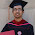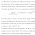### leetcode Question 73: Populating Next Right Pointers in Each Node II

Populating Next Right Pointers in Each Node II

Follow up for problem "Populating Next Right Pointers in Each Node".
What if the given tree could be any binary tree? Would your previous solution still work?
Note:
• You may only use constant extra space.
For example,
Given the following binary tree,
         1
/  \
2    3
/ \    \
4   5    7

After calling your function, the tree should look like:
         1 -> NULL
/  \
2 -> 3 -> NULL
/ \    \
4-> 5 -> 7 -> NULL

Idea is to use dfs and only constant space is used. The key is to use the "next" pointer and search right child first then the left child. Details see the code comment.

Code:
/**
* Definition for binary tree with next pointer.
* struct TreeLinkNode {
*  int val;
*  TreeLinkNode *left, *right, *next;
*  TreeLinkNode(int x) : val(x), left(NULL), right(NULL), next(NULL) {}
* };
*/
class Solution {
public:
if (!root){return;}  // if current node is not valid return

if (root->next==NULL){ // if current node is not in the right boundary of this level
if (root->right){root->right->next = NULL;} // if has right child, its next is null
if (root->left){root->left->next = root->right;}//if has left child, its next is its right
}else{ // if the current node has next node in its right
TreeLinkNode* p = root->next; //the pointer travle along this level
TreeLinkNode* q=NULL; // the next valid pointer in the level+1 , or NULL if not found
while (p){ //find the next valid child of root node
if (p->left){q =p->left; break;}
if (p->right){q =p->right; break;}
p=p->next;
}
if (root->right){root->right->next = q;} //set right child if exists
if (root->left && root->right ){root->left->next = root->right;}//set left if right exists
if (root->left && !root->right) {root->left->next = q;} // set left if right not exist
}

dfs(root->right); // search right child, order is important
dfs(root->left);  // search left child
}
void connect(TreeLinkNode *root) {
// Start typing your C/C++ solution below
// DO NOT write int main() function
if (!root){return;}
root->next = NULL;
dfs(root);
}
};


Old solution (BFS):
Idea is almost the same to previous problem.
Just we cannot use the level information directly, so we can store the level info!
Use a pair instead of a single tree node in the queue.
Details see the code.

Code:
/**
* Definition for binary tree with next pointer.
* struct TreeLinkNode {
*  int val;
*  TreeLinkNode *left, *right, *next;
*  TreeLinkNode(int x) : val(x), left(NULL), right(NULL), next(NULL) {}
* };
*/
class Solution {
public:
void connect(TreeLinkNode *root) {
// Start typing your C/C++ solution below
// DO NOT write int main() function
queue<pair<TreeLinkNode*, int> > que;
if (root==NULL) {return;}

while (!que.empty()){
que.pop();
if (p.first->left!=NULL){
}
if (p.first->right!=NULL){
}

if (que.empty()){
p.first->next = NULL;
return;
}
if (p.second != que.front().second){
p.first->next = NULL;
}else{
p.first->next = que.front().first;
}
}

}
};


1.1.